# Perturbed orbit in standard reference frame

Let (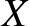,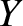,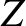) be Cartesian coordinates in the standard reference frame described in Section 4.12. The relationship between the (,,) and the (,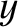,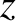) coordinate systems is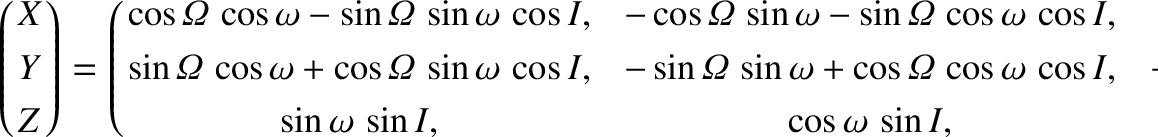(I.33)

Here,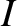,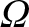, and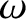are the planet's orbital inclination to the ecliptic plane, longitude of the ascending node (relative to the vernal equinox), and argument of the perihelion, respectively.

Equations (I.6) and (I.33) imply that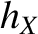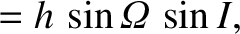(I.34)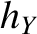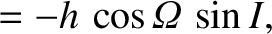(I.35)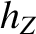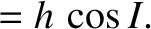(I.36)

It follows that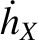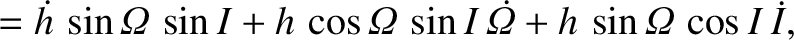(I.37)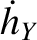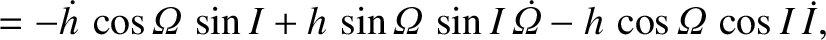(I.38)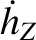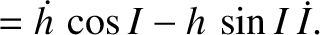(I.39)

However, Equations (I.19)–(I.21) can be combined with Equation (I.33) to give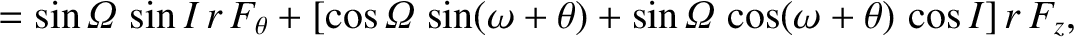(I.40)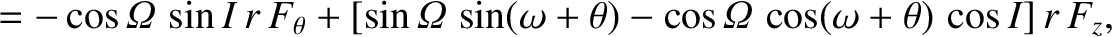(I.41)(I.42)

Thus, Equations (I.22), (I.39), and (I.42) yield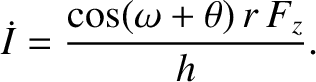(I.43)

Equations (I.34), (I.35), (I.37), and (I.38) imply that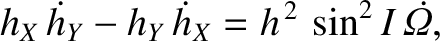(I.44)

or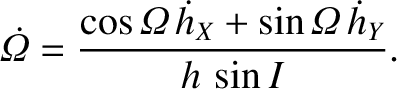(I.45)

However, it follows from Equations (I.40) and (I.41) that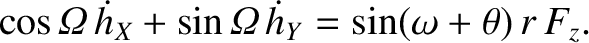(I.46)

Hence, we obtain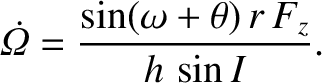(I.47)

According to Equations (I.8) and (I.33),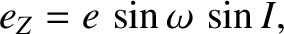(I.48)

which implies that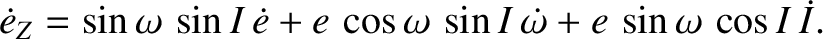(I.49)

Thus, it follows from Equations (I.27) and (I.43) that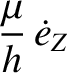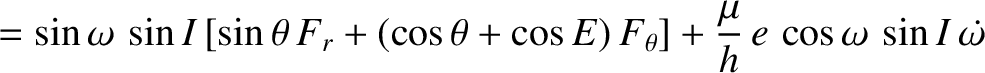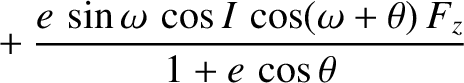(I.50)

However, Equations (I.24)–(I.26) and (I.33) give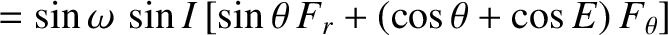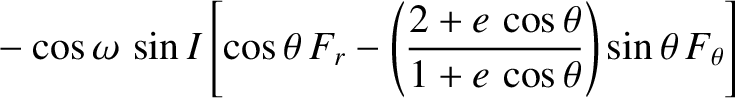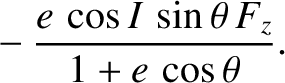(I.51)

A comparison of the previous two equations yields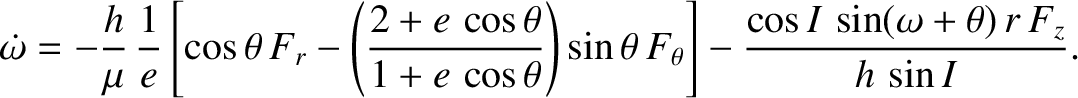(I.52)